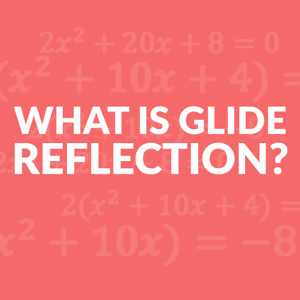# Definition, Math Example, Formula Calculation

Geometry Lesson 63: Glide Reflections
Geometry Lesson 63: Glide Reflections

##What is Glide Reflection?

Contents

Definition: A glide reflection in math is a combination of transformations in 2-dimensional geometry. First, a translation is performed on the figure, and then it is reflected over a line. Therefore, Glide reflection is also known as trans-flection.

Translation simply means moving, every point of the shape must move the same distance, and in the same direction.

Reflection means reflecting an image over a mirror line. Every point is the same distance from the central line after performing reflection on an object.

### Characteristics:

Glide reflection is the composition of translation and a reflection, where the translation is parallel to the line of reflection or reflection in line parallel to the direction of translation. A glide reflection is – commutative and have opposite isometry.

### Uses:

Glide reflections are essential to an analysis of symmetries.

### How it occurs:

Glide reflection occurs when you perform translation (glide) on a figure and followed by a reflection across a line parallel to the direction of translation.

### Commutative properties:

A glide refection is commutative. Outcome will not affect if you reverse the composition of transformation performed on the figure. Whether you perform translation first and followed by reflection or you perform reflection first and followed by translation, outcome remains same.

For example, foot prints. Look at our example of this concept below.

## Glide Reflection & Opposite isometry

An opposite isometry preserves the distance but orientation changes, from clockwise to anti-clockwise (counter clockwise) or from anti-clockwise(counter clockwise) to clockwise.

Reflection transformation is an opposite isometry, and therefore every glide reflection is also an opposite isometry. Distance remains preserved but orientation (or order) changes in a glide reflection. From the four types of transformations translation, reflection, glide reflection, and rotation.

Reflection and glide reflection are opposite isometry.

## Properties preserved (invariant) under a glide reflection

Following properties remain preserved in translation and reflection; therefore also remain preserved in a glide reflection.

• Distance is preserved in a glide reflection.
• Angle measure is preserved in a glide reflection.
• Parallelism is preserved in a glide reflection.
• Perpendicularity is preserved in a glide reflection.
• Collinearity (points stay on the same lines) is preserved in a glide reflection.
• Midpoint (midpoints remains the same in each figure) is preserved in a glide reflection.

## Glide Reflection Formula

Glide reflection is a composition of translation and reflection. Therefore, we have to use translation rule and reflection rule to perform a glide reflection on a figure.

1) Translation rule

• Right h units (x, y) → (x + h, y).
• Left h units (x, y) → (x – h, y).
• Up k units (x, y) → (x, y + k).
• Down k units (x, y) → (x, y – k).

2) A line to reflect over

• Reflection in x-axis: (x, y) → (x, -y)
• Reflection in y-axis: (x, y) → (-x, y)
• Reflection in y = x: (x, y) → (y, x)
• Reflection in y = -x: (x, y) → (-y, -x)

## Glide Reflection Example Calculation

In everyday life, a classic example of glide reflection is the track of footprints left in the sand by a person walking over it.

In the above diagram, translation (glide) performed on the foot, and then reflection across the parallel line of translation, then again glide followed by the refection, this foot steps are the typical example of glide reflection.

In the above diagram, PQR is a triangle with coordinates of the vertices P(-1, -3), Q(-4, -1), and R(-6, -4). It is translated 10 units right.

• Right ‘h’ units (x, y) → (x + h, y)
• P(-1, -3) → P’(-1 + 10, -3) = P’(9, -3)
• Q(-4, -1) → Q’(-4 + 10, -1) = Q’(6, -1)
• R(-6, -4) → R’(-6 + 10, -4) = R’(4, -4)

Therefore the coordinates of the triangle P’Q’R’ are P’(9, -3), Q’(6, -1) and R’(4, -4).

Reflection over the x-axis is performed on the triangle P’Q’R’.

• Reflection in x-axis: (x, y) → (x, -y)
• P’(9, -3) → P’’(9, 3)
• Q’(6, -1) → Q’’(6, 1)
• R’(4, -4) → R’’(4, 4)

Triangle P’’Q’’R’’ is an outcome of glide translation performed on the triangle PQR.

Glide reflection is commutative, whether we glide first then reflect or we reflect first and then glide, outcome remains same.

In the diagram shown above, in the left side of transformation, reflection is performed on the blue pentagon first, outcome is the red pentagon and then translation is performed on the red pentagon and outcome is the green pentagon.

On the right side of diagram, translation is performed on the blue pentagon first, outcome is the red pentagon and then reflection is performed on the red pentagon and outcome is the green pentagon. It is clear from the diagram, that the final outcome is the same in every aspect. In Glide reflection, reversing the direction of the composition will not affect the outcome.

You are watching: Definition, Math Example, Formula Calculation. Info created by THVinhTuy selection and synthesis along with other related topics.

Rate this post